# NCERT Exemplar Solutions for Class 7 Maths Chapter 4 Simple Equations

NCERT Exemplar Solutions for Class 7 Maths Chapter 4 Simple Equations are given here in a simple but comprehensive way. Simple Equations solutions are extremely helpful for students to clear all their doubts easily and understand the basics of the chapter more effectively. It is essential to understand the various kinds of questions and figure out the best answers for them. Students find NCERT Exemplar Solutions for Class 7 Maths Chapter 4, where they can understand completely about the equations.

Simple Equations can be defined as a mathematical statement consisting of an equal symbol between two algebraic expressions that have the same value. Topics covered in NCERT Exemplar Solutions for Class 7 Maths Chapter 4 are transposing, solutions to equations and applications of simple equations to practical situations.

## Download the PDF of NCERT Exemplar Solutions for Class 7 Maths Chapter 4 Simple Equations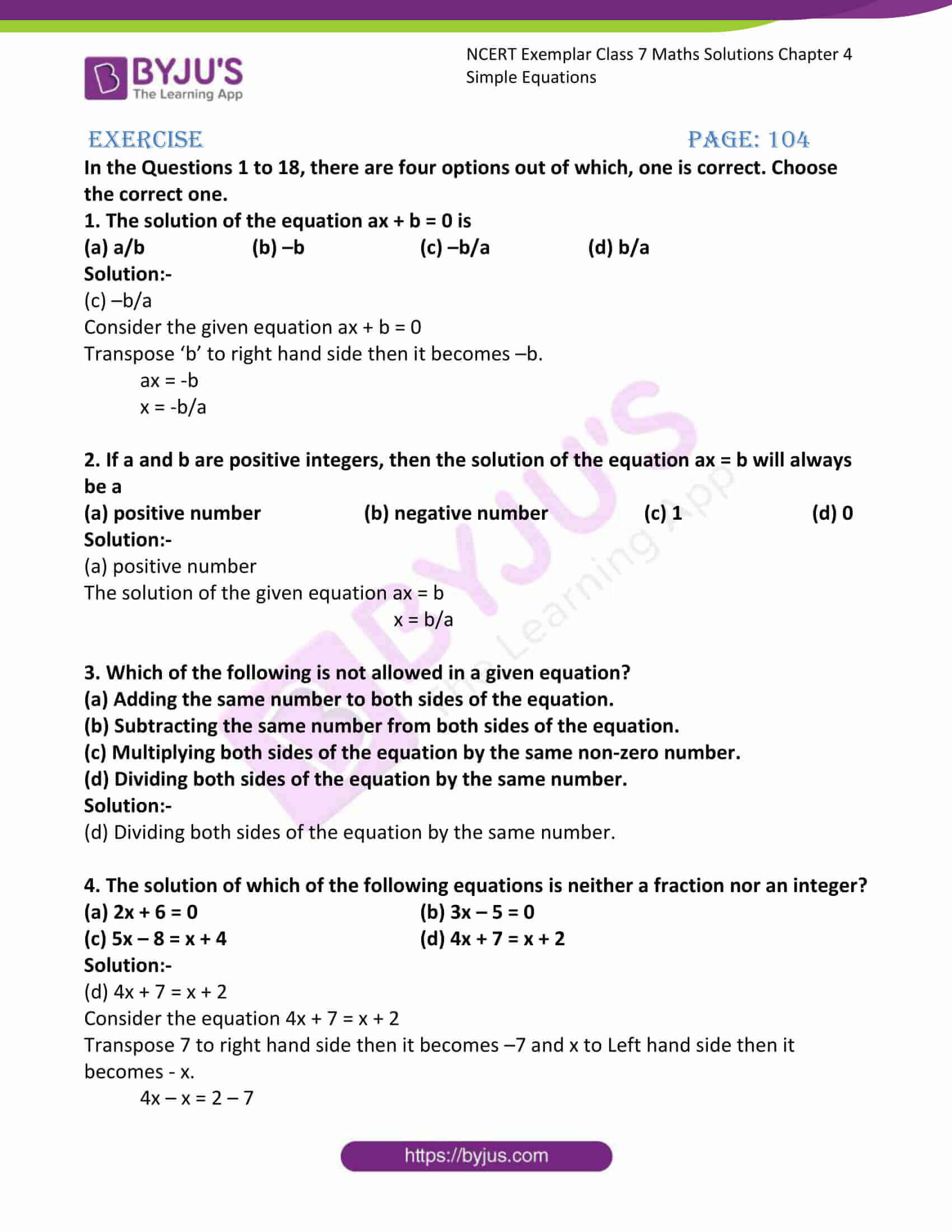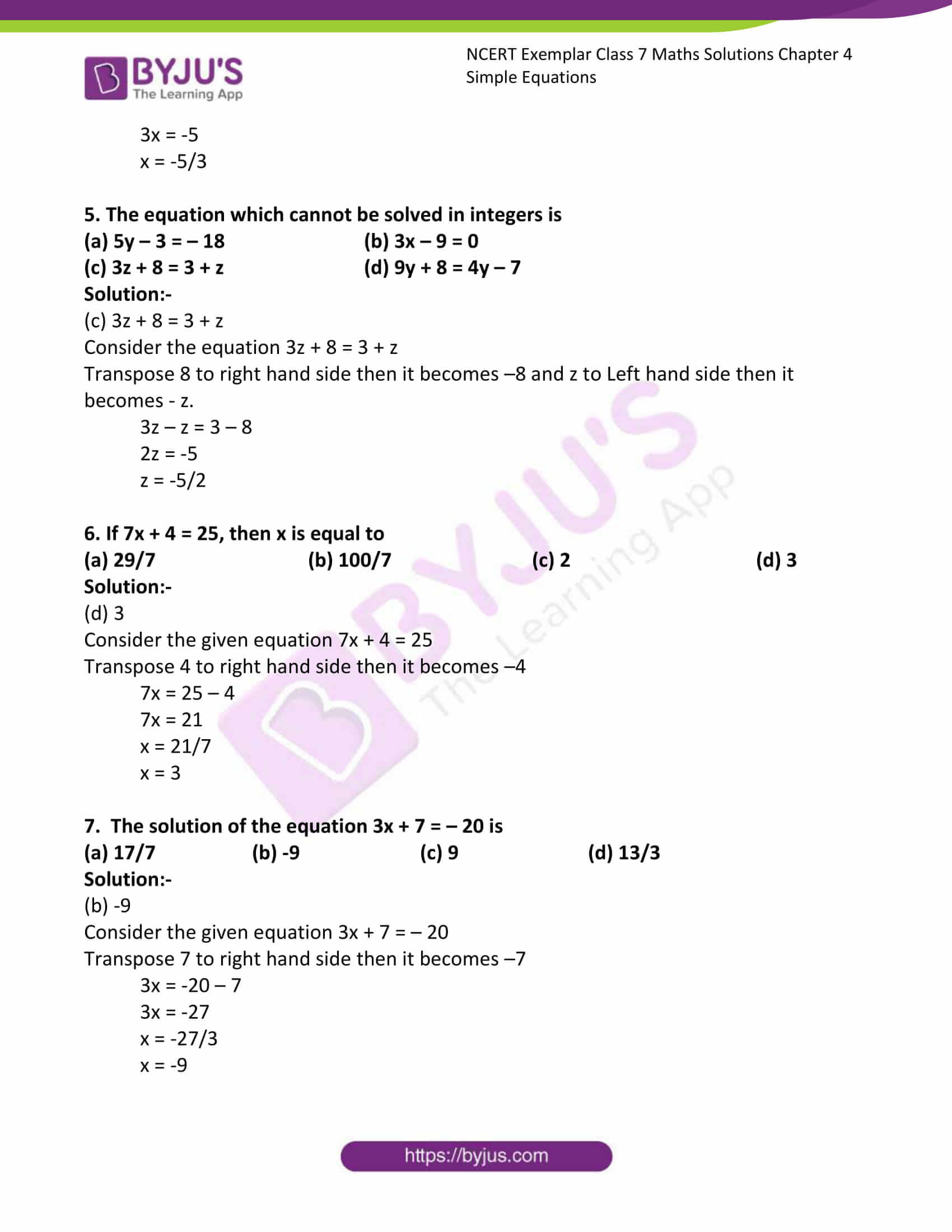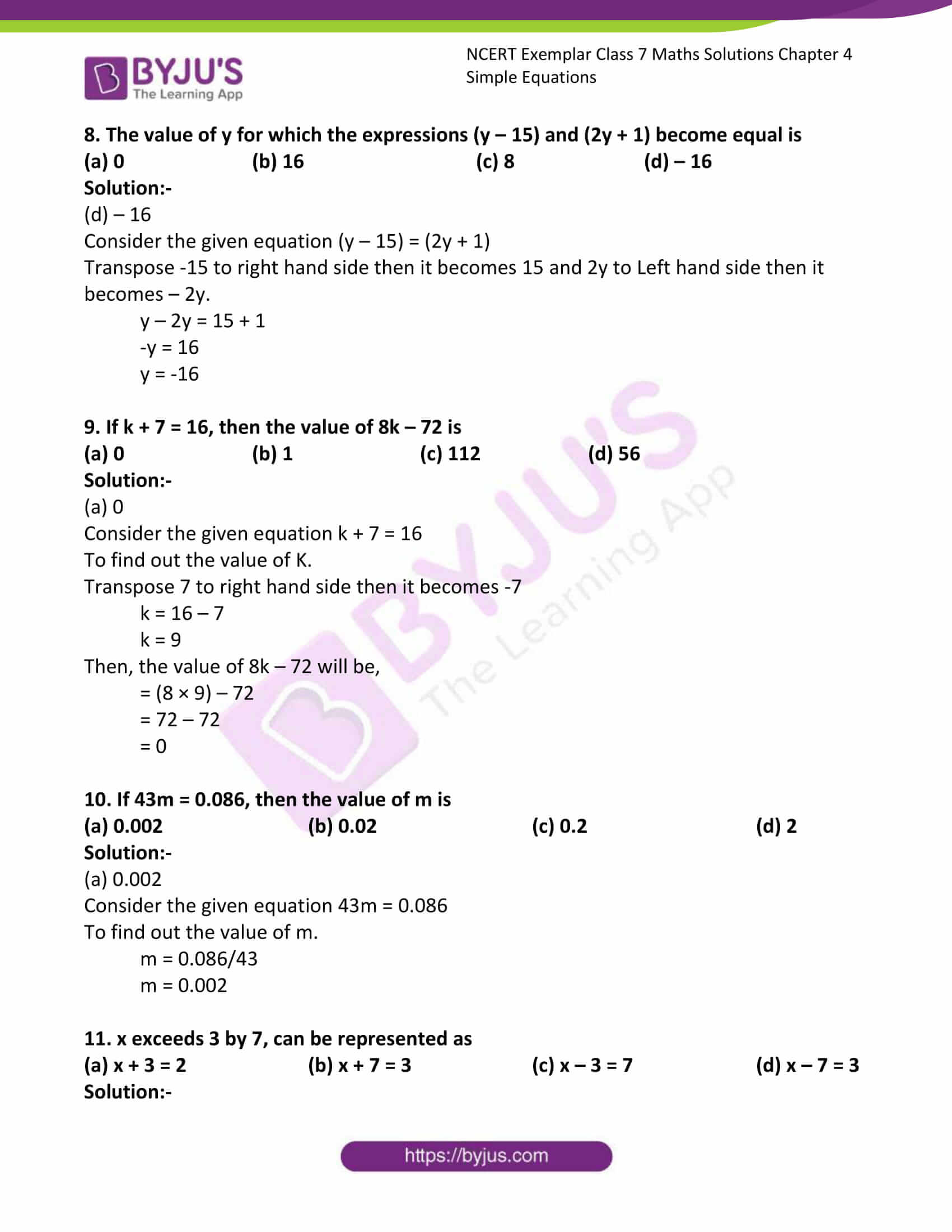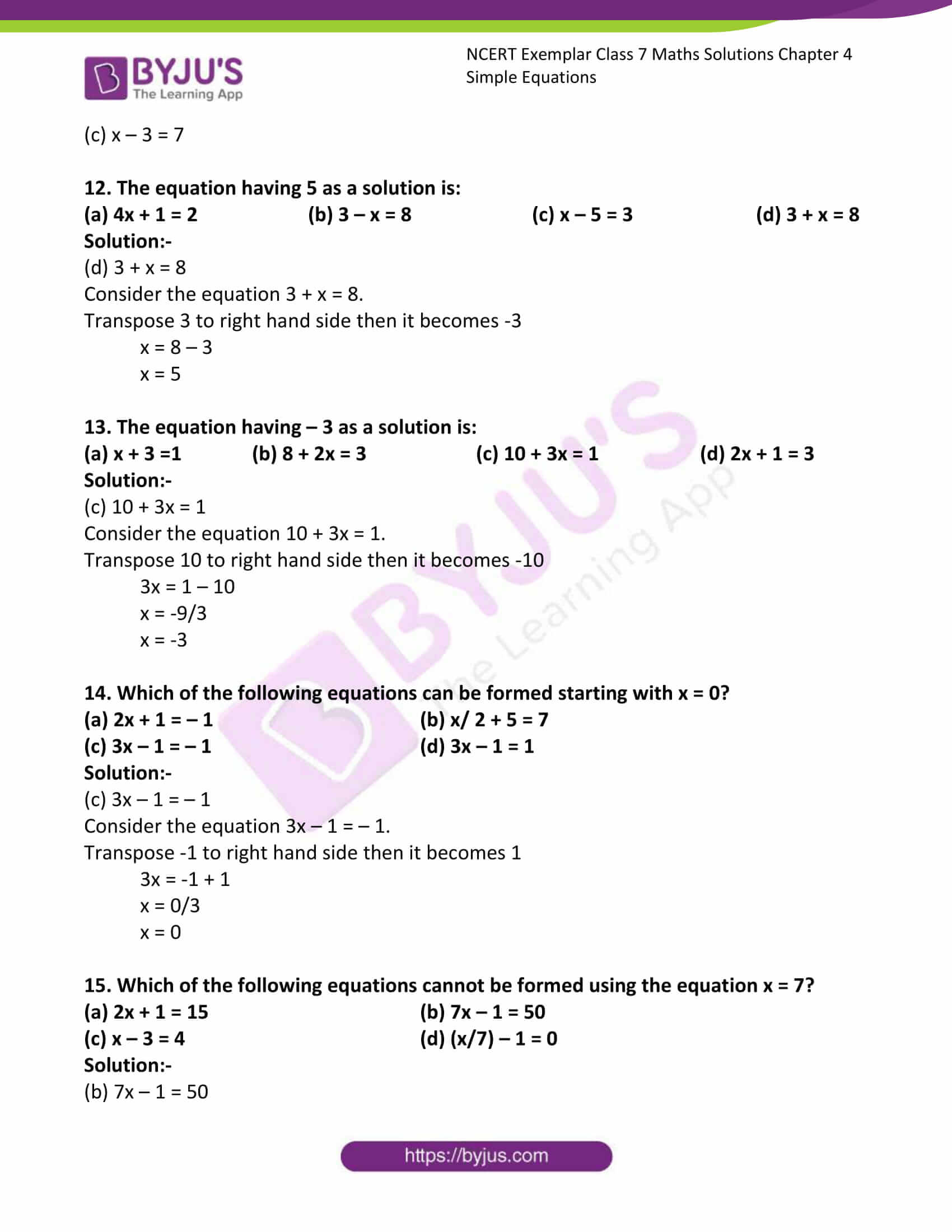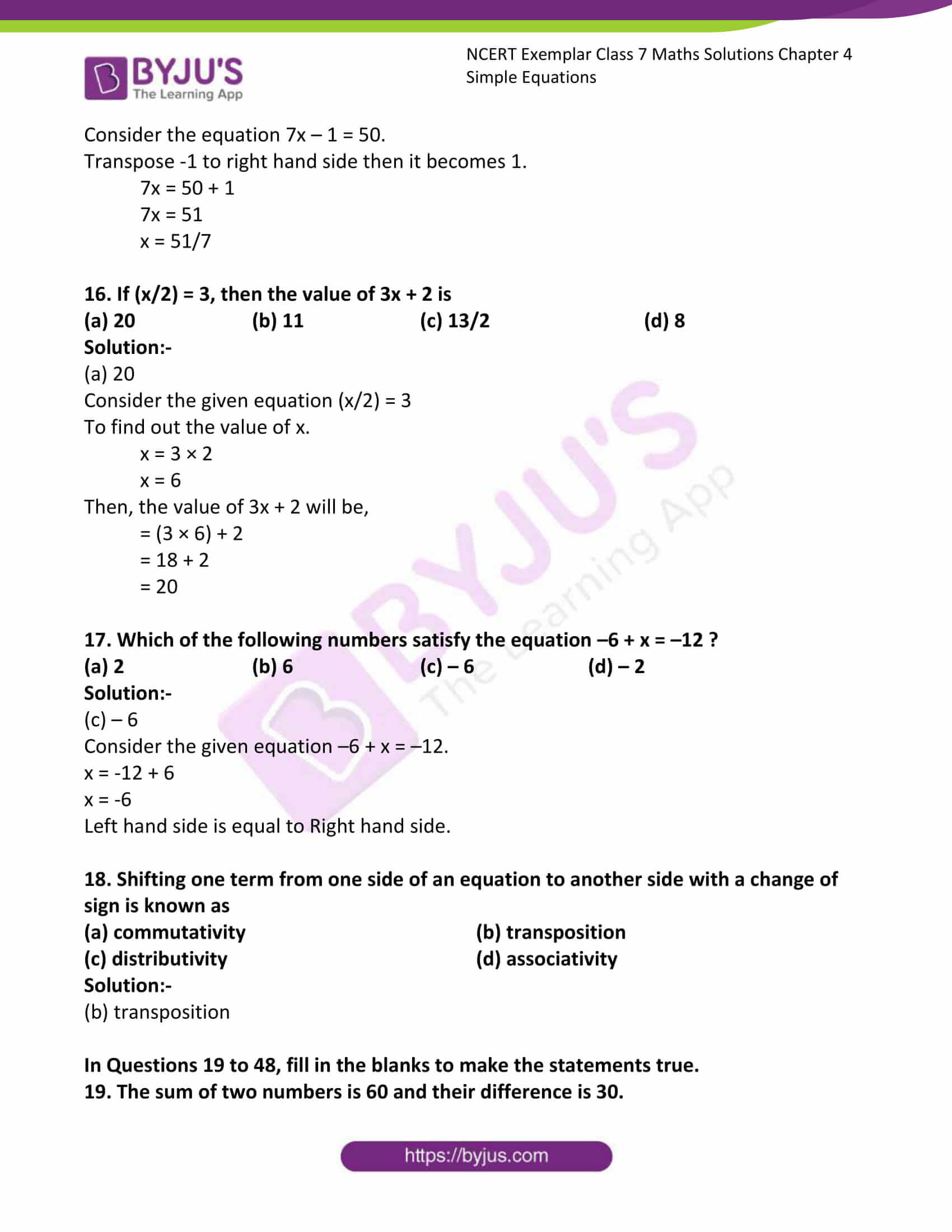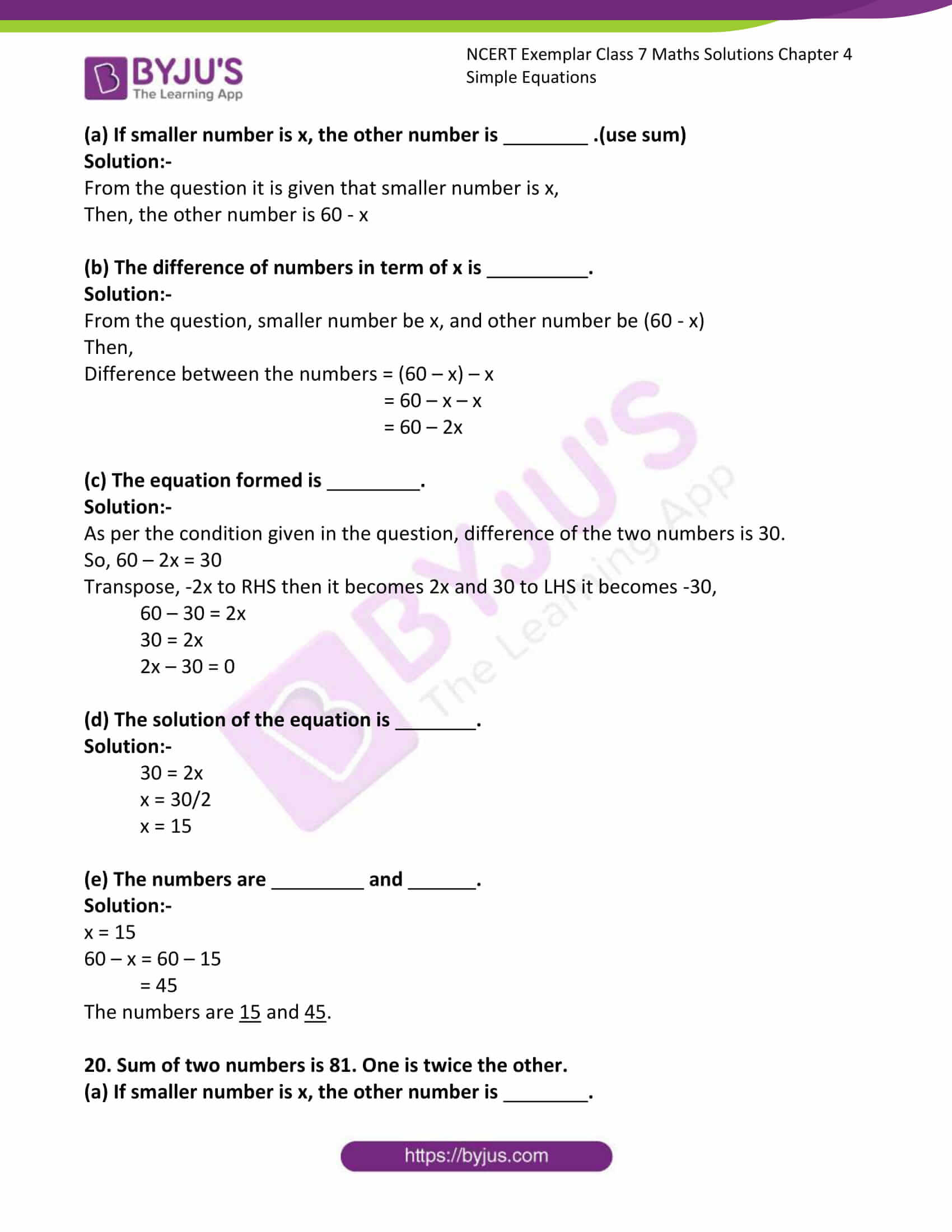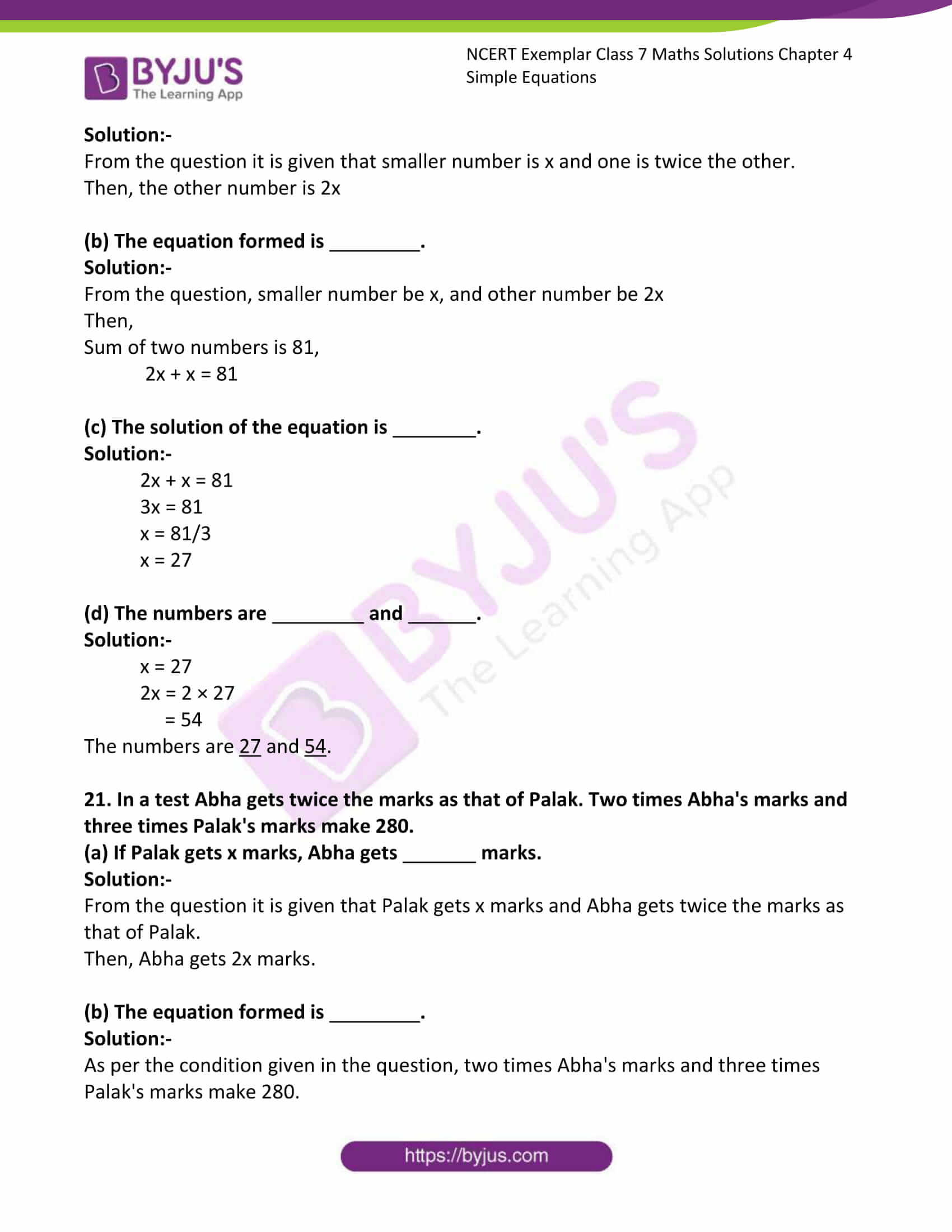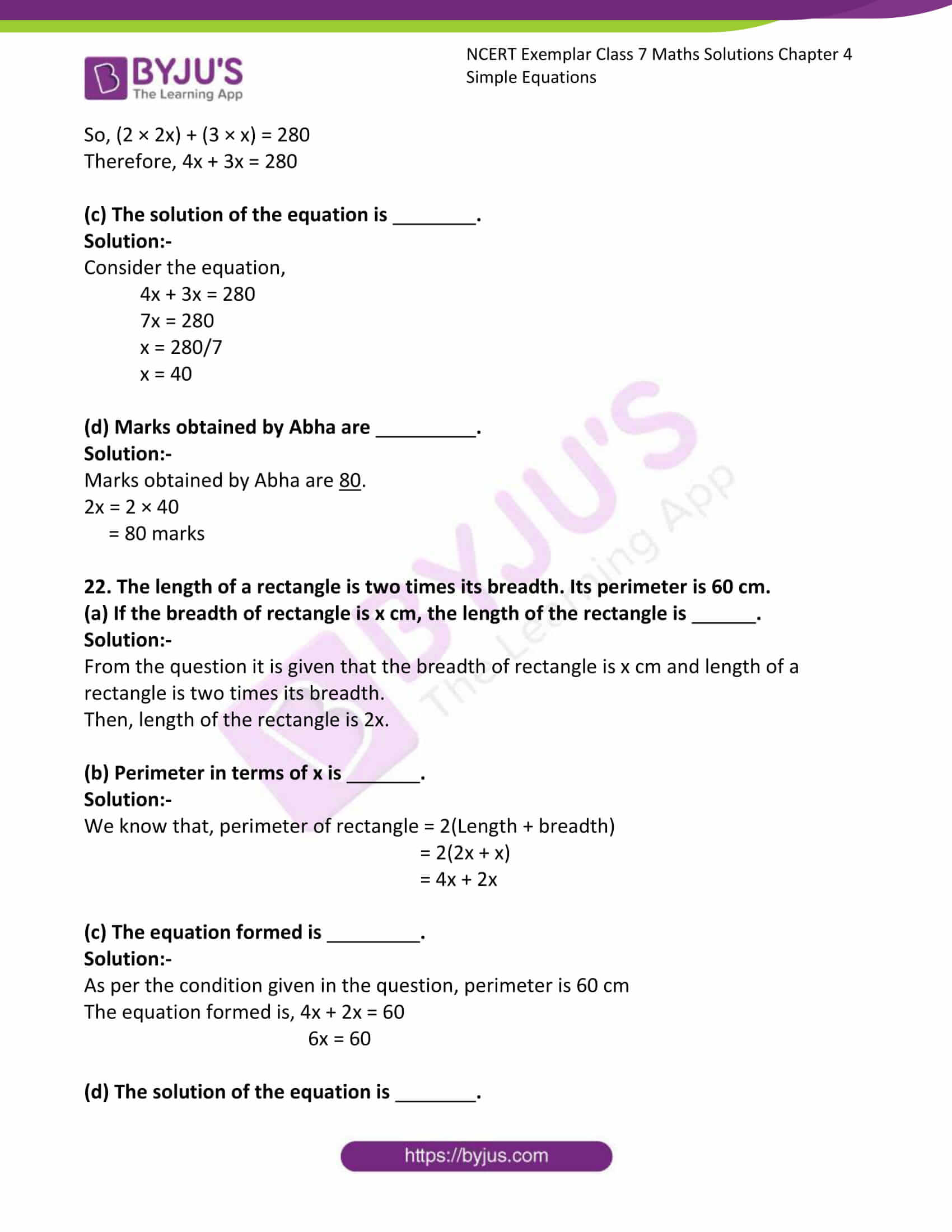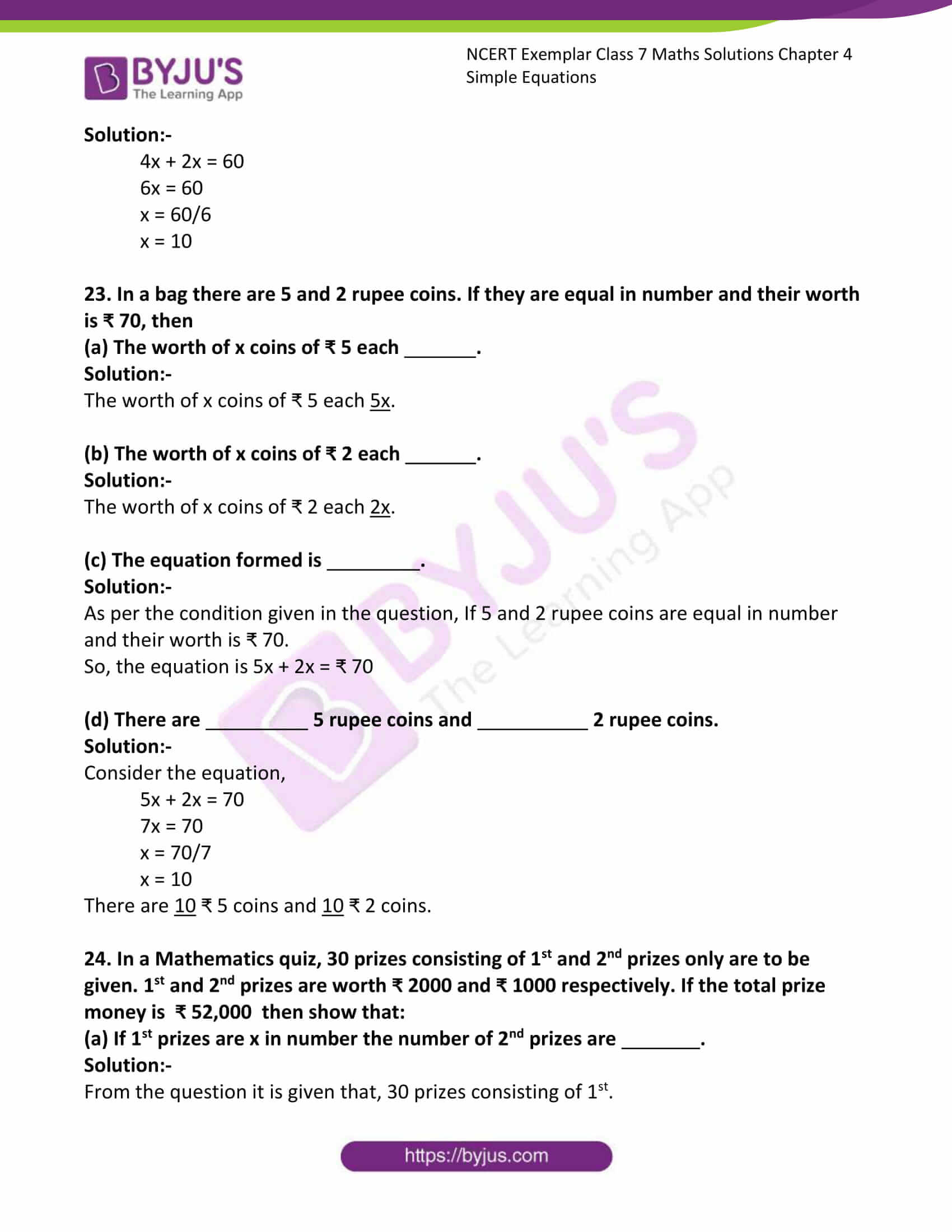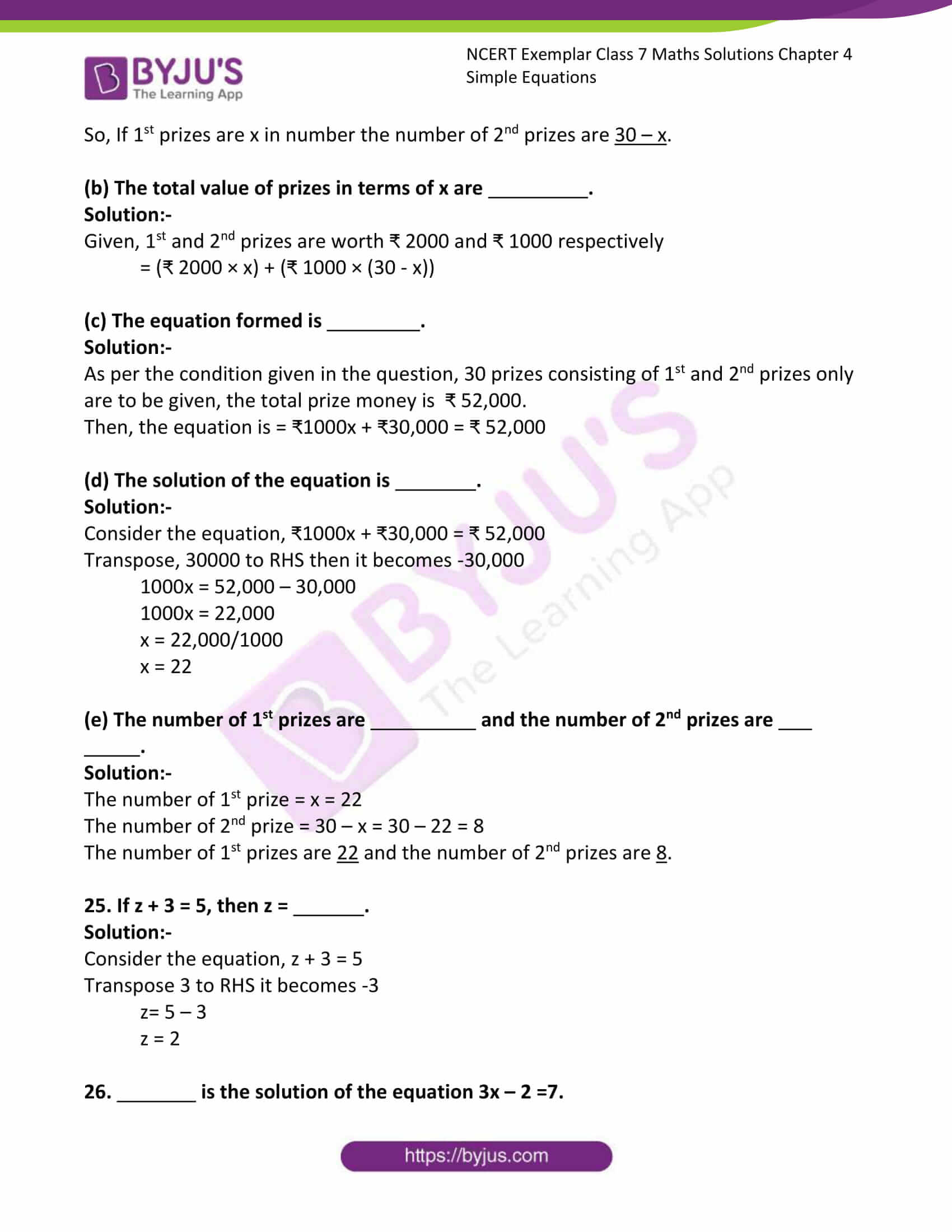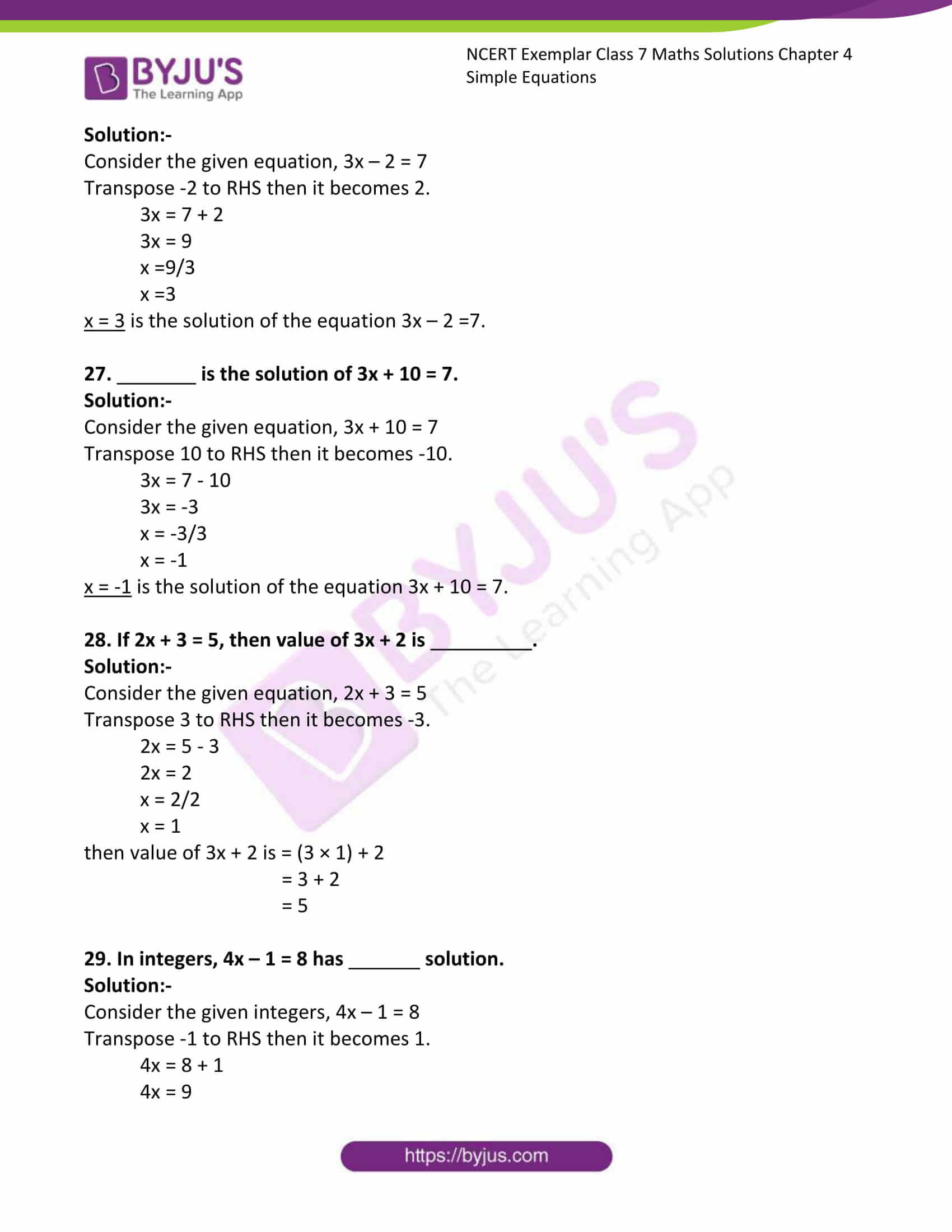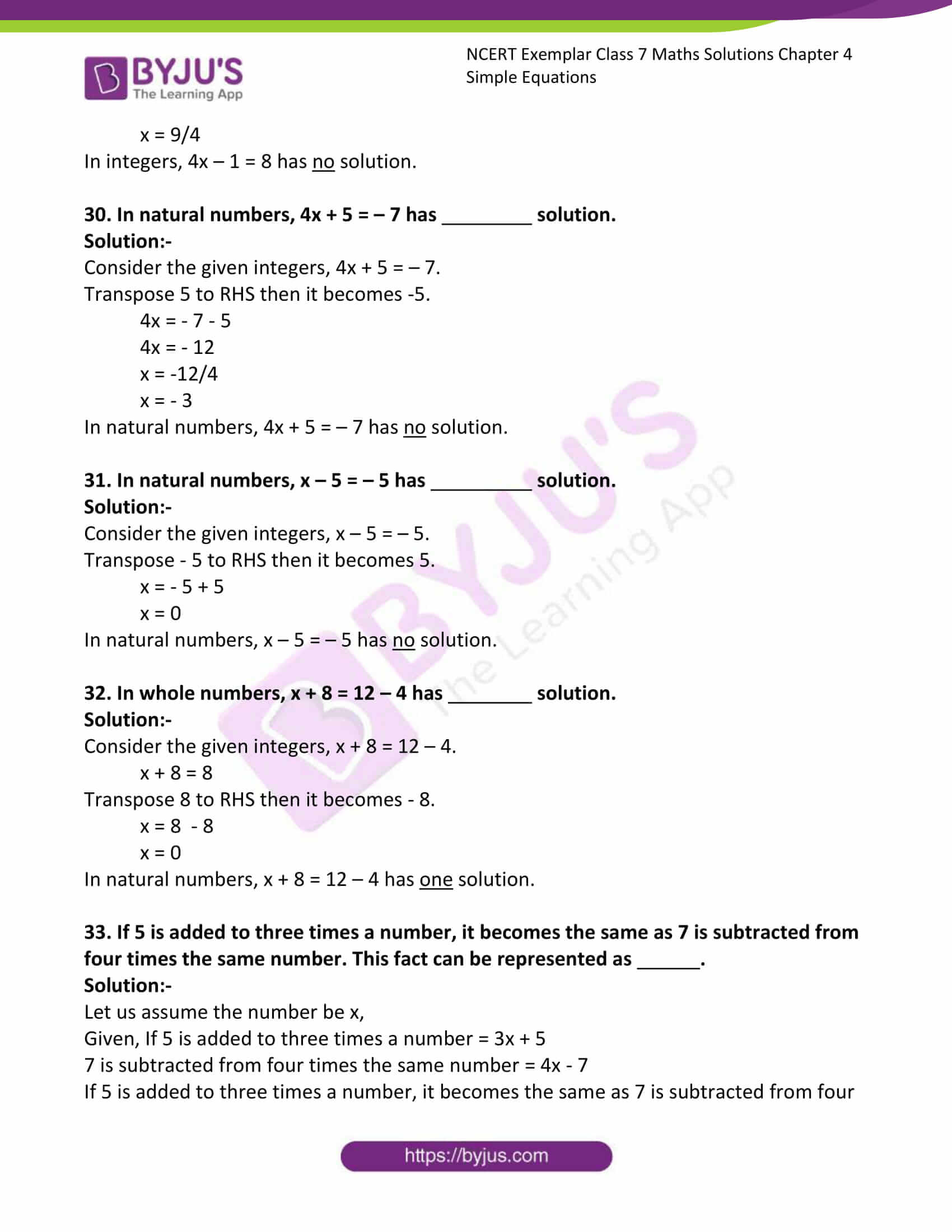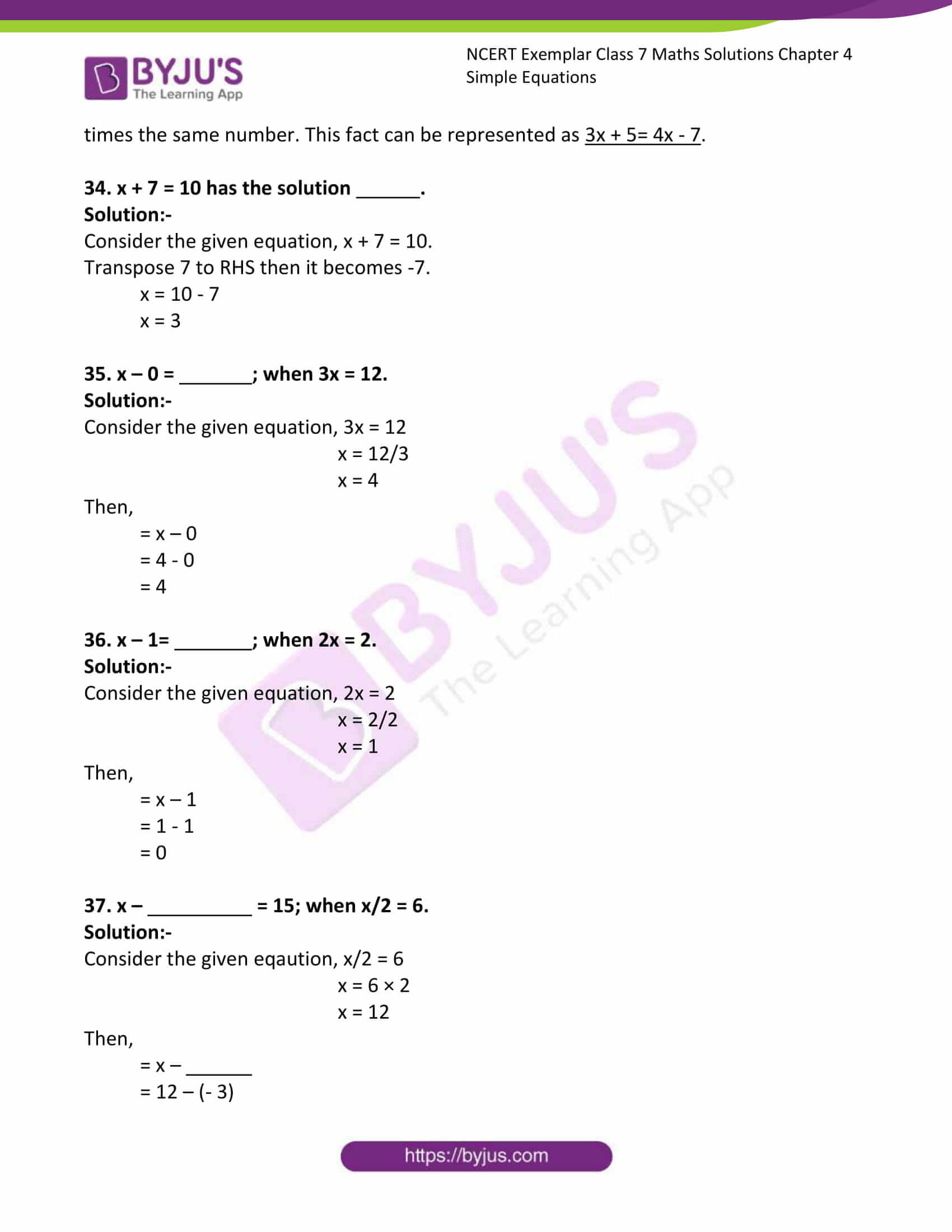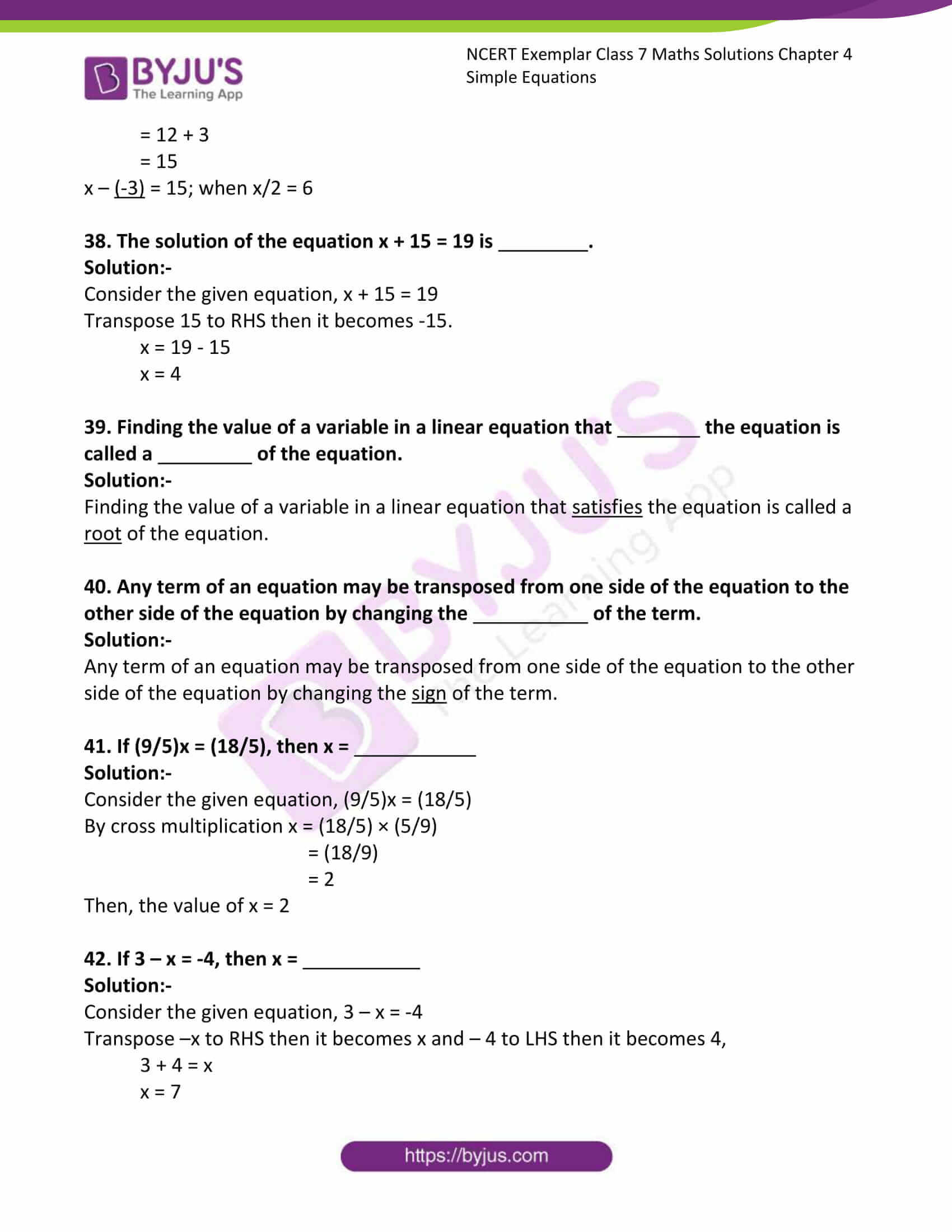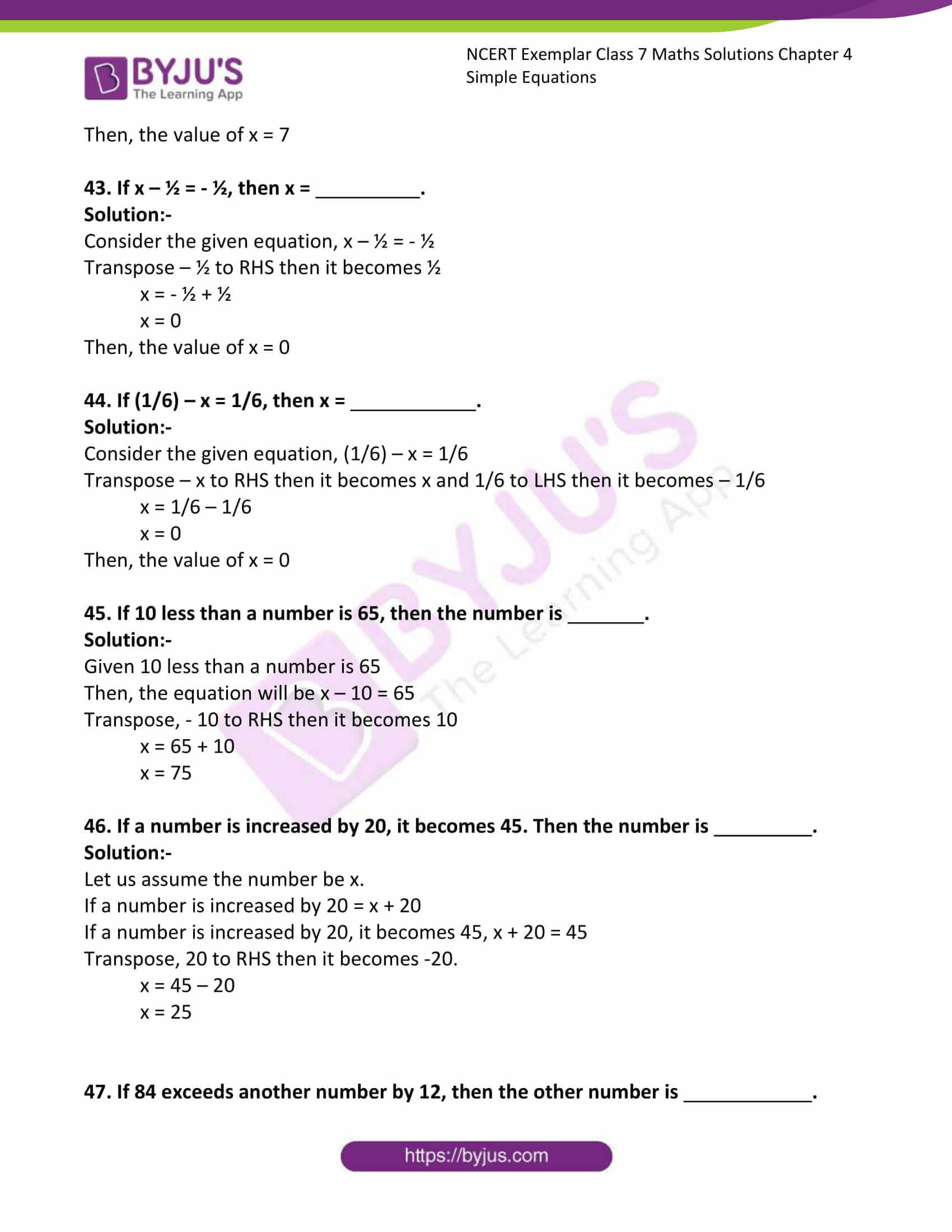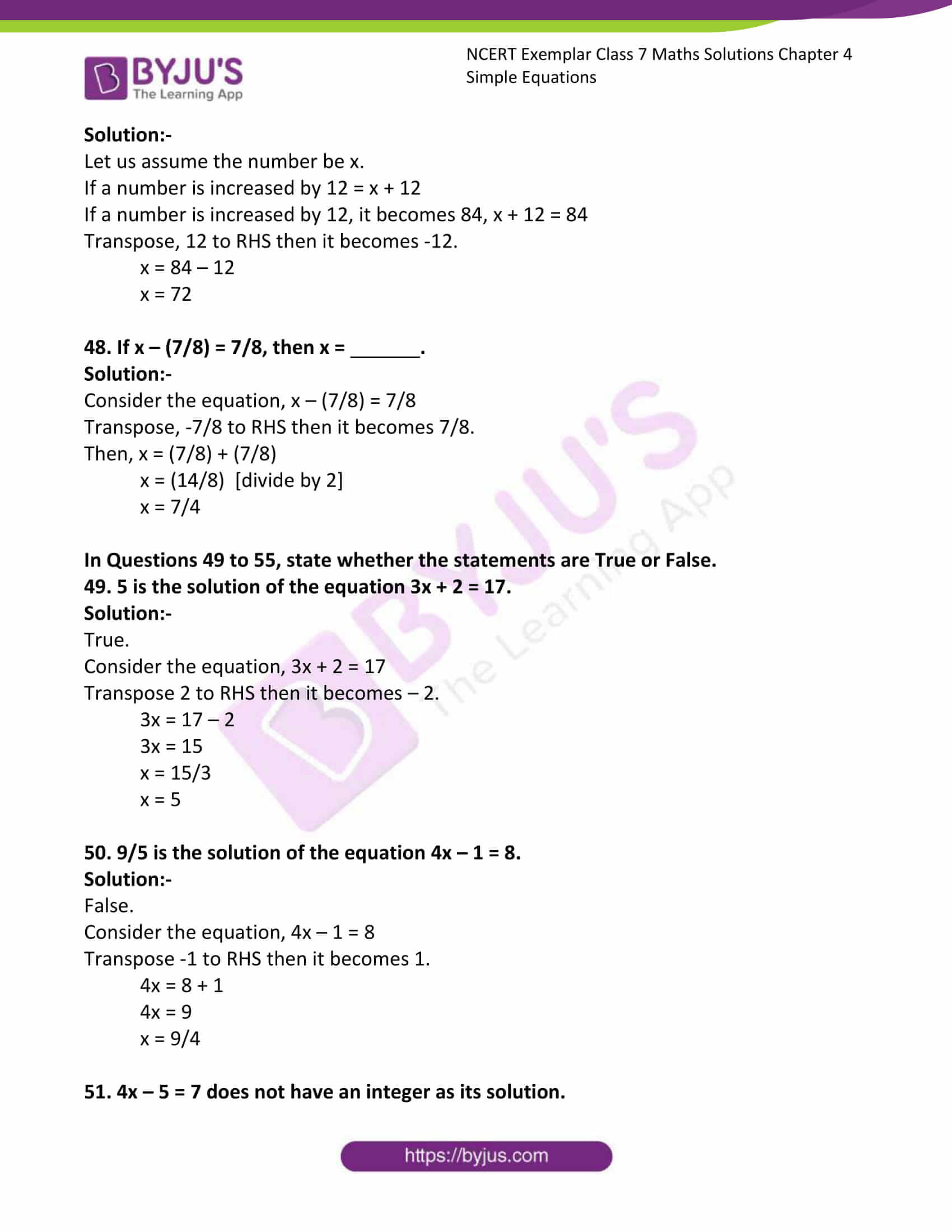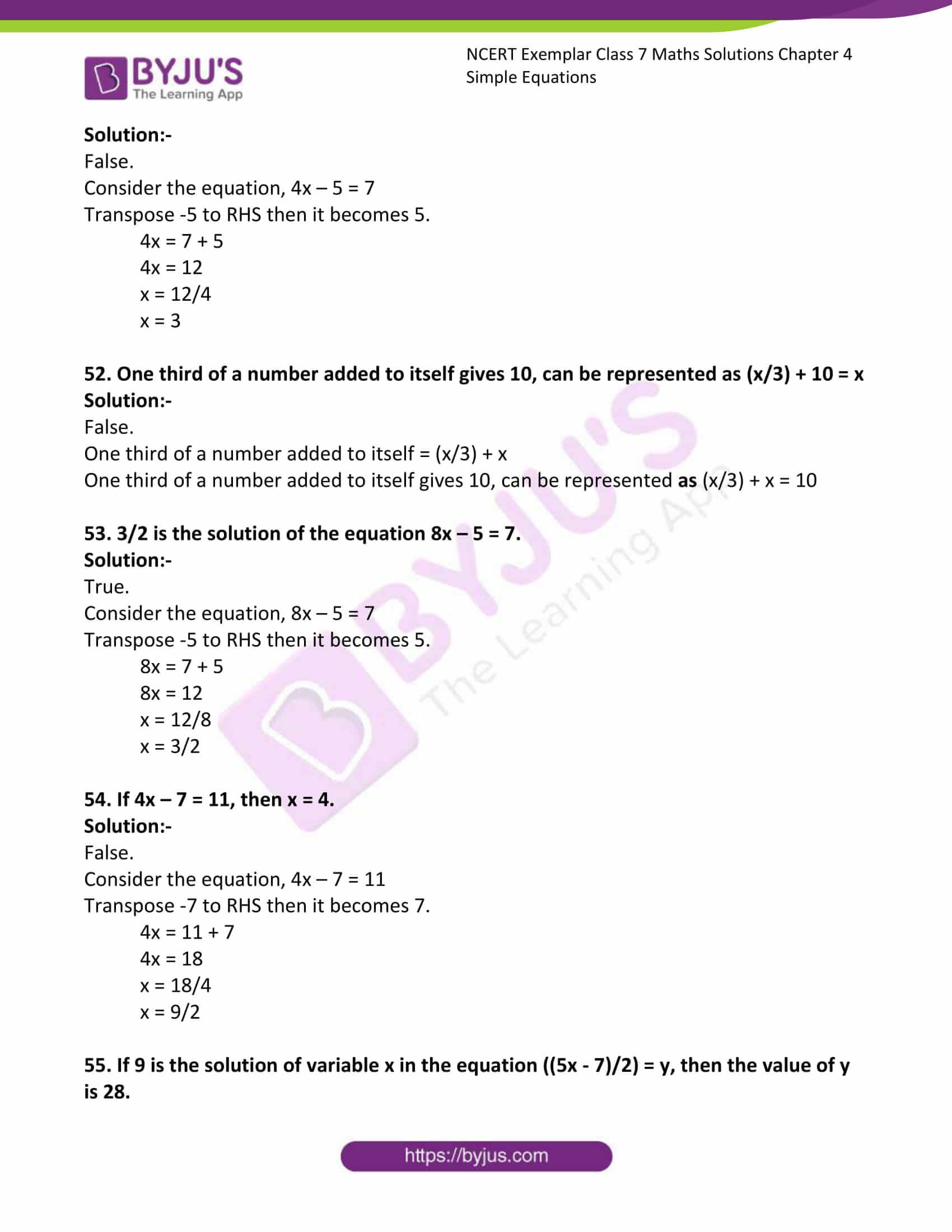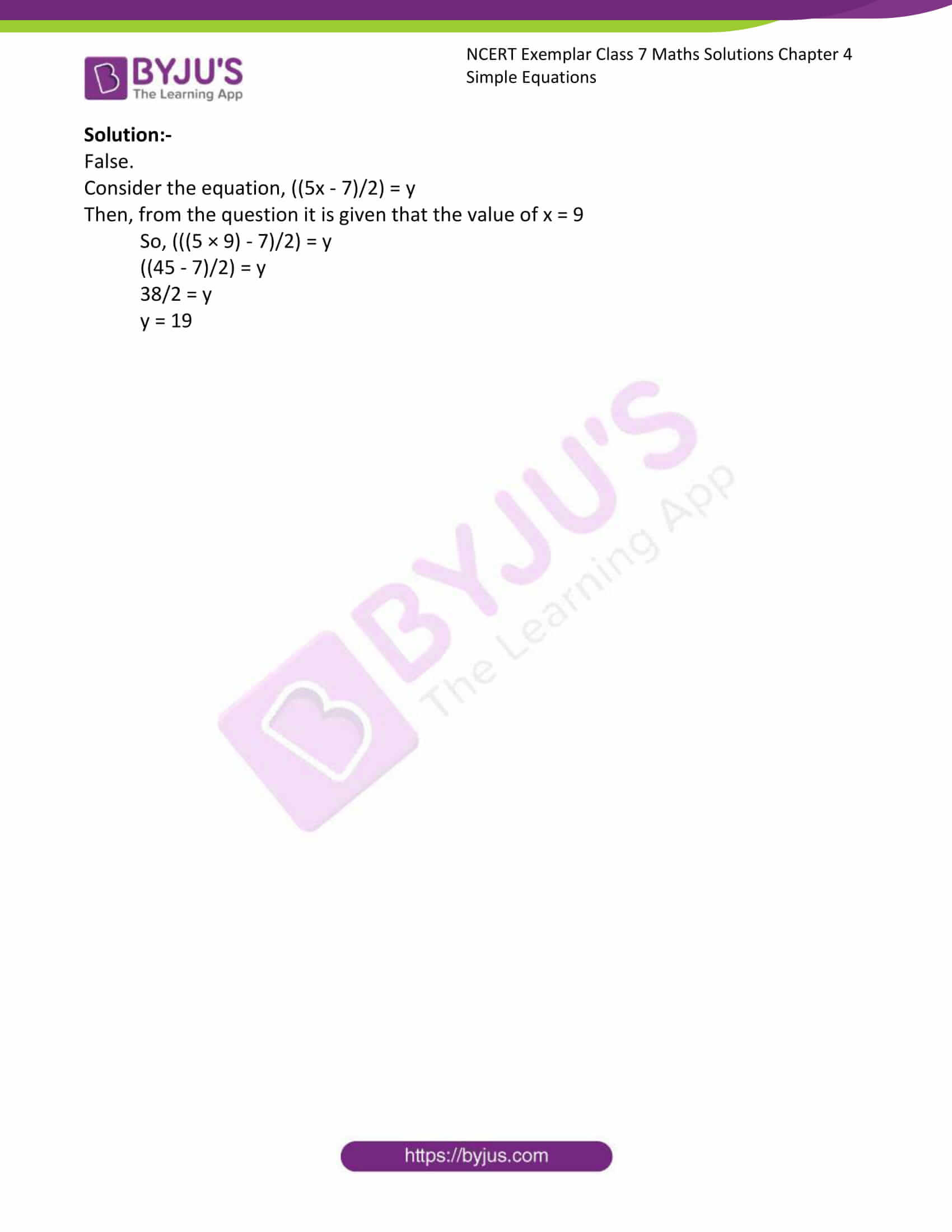Exercise Page: 104

In the Questions 1 to 18, there are four options out of which, one is correct. Choose the correct one.

1. The solution of the equation ax + b = 0 is

(a) a/b (b) –b (c) –b/a (d) b/a

Solution:-

(c) –b/a

Consider the given equation ax + b = 0

Transpose ‘b’ to right hand side then it becomes –b.

ax = -b

x = -b/a

2. If a and b are positive integers, then the solution of the equation ax = b will always be a

(a) positive number (b) negative number (c) 1 (d) 0

Solution:-

(a) positive number

The solution of the given equation ax = b

x = b/a

3. Which of the following is not allowed in a given equation?

(a) Adding the same number to both sides of the equation.

(b) Subtracting the same number from both sides of the equation.

(c) Multiplying both sides of the equation by the same non-zero number.

(d) Dividing both sides of the equation by the same number.

Solution:-

(d) Dividing both sides of the equation by the same number.

4. The solution of which of the following equations is neither a fraction nor an integer? (a) 2x + 6 = 0 (b) 3x – 5 = 0

(c) 5x – 8 = x + 4 (d) 4x + 7 = x + 2

Solution:-

(d) 4x + 7 = x + 2

Consider the equation 4x + 7 = x + 2

Transpose 7 to right hand side then it becomes –7 and x to Left hand side then it becomes – x.

4x – x = 2 – 7

3x = -5

x = -5/3

5. The equation which cannot be solved in integers is

(a) 5y – 3 = – 18 (b) 3x – 9 = 0

(c) 3z + 8 = 3 + z (d) 9y + 8 = 4y – 7

Solution:-

(c) 3z + 8 = 3 + z

Consider the equation 3z + 8 = 3 + z

Transpose 8 to right hand side then it becomes –8 and z to Left hand side then it becomes – z.

3z – z = 3 – 8

2z = -5

z = -5/2

6. If 7x + 4 = 25, then x is equal to

(a) 29/7 (b) 100/7 (c) 2 (d) 3

Solution:-

(d) 3

Consider the given equation 7x + 4 = 25

Transpose 4 to right hand side then it becomes –4

7x = 25 – 4

7x = 21

x = 21/7

x = 3

7. The solution of the equation 3x + 7 = – 20 is

(a) 17/7 (b) -9 (c) 9 (d) 13/3

Solution:-

(b) -9

Consider the given equation 3x + 7 = – 20

Transpose 7 to right hand side then it becomes –7

3x = -20 – 7

3x = -27

x = -27/3

x = -9

8. The value of y for which the expressions (y – 15) and (2y + 1) become equal is

(a) 0 (b) 16 (c) 8 (d) – 16

Solution:-

(d) – 16

Consider the given equation (y – 15) = (2y + 1)

Transpose -15 to right hand side then it becomes 15 and 2y to Left hand side then it becomes – 2y.

y – 2y = 15 + 1

-y = 16

y = -16

9. If k + 7 = 16, then the value of 8k – 72 is

(a) 0 (b) 1 (c) 112 (d) 56

Solution:-

(a) 0

Consider the given equation k + 7 = 16

To find out the value of K.

Transpose 7 to right hand side then it becomes -7

k = 16 – 7

k = 9

Then, the value of 8k – 72 will be,

= (8 × 9) – 72

= 72 – 72

= 0

10. If 43m = 0.086, then the value of m is

(a) 0.002 (b) 0.02 (c) 0.2 (d) 2

Solution:-

(a) 0.002

Consider the given equation 43m = 0.086

To find out the value of m.

m = 0.086/43

m = 0.002

11. x exceeds 3 by 7, can be represented as

(a) x + 3 = 2 (b) x + 7 = 3 (c) x – 3 = 7 (d) x – 7 = 3

Solution:-

(c) x – 3 = 7

12. The equation having 5 as a solution is:

(a) 4x + 1 = 2 (b) 3 – x = 8 (c) x – 5 = 3 (d) 3 + x = 8

Solution:-

(d) 3 + x = 8

Consider the equation 3 + x = 8.

Transpose 3 to right hand side then it becomes -3

x = 8 – 3

x = 5

13. The equation having – 3 as a solution is:

(a) x + 3 =1 (b) 8 + 2x = 3 (c) 10 + 3x = 1 (d) 2x + 1 = 3

Solution:-

(c) 10 + 3x = 1

Consider the equation 10 + 3x = 1.

Transpose 10 to right hand side then it becomes -10

3x = 1 – 10

x = -9/3

x = -3

14. Which of the following equations can be formed starting with x = 0?

(a) 2x + 1 = – 1 (b) x/ 2 + 5 = 7

(c) 3x – 1 = – 1 (d) 3x – 1 = 1

Solution:-

(c) 3x – 1 = – 1

Consider the equation 3x – 1 = – 1.

Transpose -1 to right hand side then it becomes 1

3x = -1 + 1

x = 0/3

x = 0

15. Which of the following equations cannot be formed using the equation x = 7?

(a) 2x + 1 = 15 (b) 7x – 1 = 50

(c) x – 3 = 4 (d) (x/7) – 1 = 0

Solution:-

(b) 7x – 1 = 50

Consider the equation 7x – 1 = 50.

Transpose -1 to right hand side then it becomes 1.

7x = 50 + 1

7x = 51

x = 51/7

16. If (x/2) = 3, then the value of 3x + 2 is

(a) 20 (b) 11 (c) 13/2 (d) 8

Solution:-

(a) 20

Consider the given equation (x/2) = 3

To find out the value of x.

x = 3 × 2

x = 6

Then, the value of 3x + 2 will be,

= (3 × 6) + 2

= 18 + 2

= 20

17. Which of the following numbers satisfy the equation –6 + x = –12 ?

(a) 2 (b) 6 (c) – 6 (d) – 2

Solution:-

(c) – 6

Consider the given equation –6 + x = –12.

x = -12 + 6

x = -6

Left hand side is equal to Right hand side.

18. Shifting one term from one side of an equation to another side with a change of sign is known as

(a) commutativity (b) transposition

(c) distributivity (d) associativity

Solution:-

(b) transposition

In Questions 19 to 48, fill in the blanks to make the statements true.

19. The sum of two numbers is 60 and their difference is 30.

(a) If smaller number is x, the other number is .(use sum)

Solution:-

From the question it is given that smaller number is x,

Then, the other number is 60 – x

(b) The difference of numbers in term of x is .

Solution:-

From the question, smaller number be x, and other number be (60 – x)

Then,

Difference between the numbers = (60 – x) – x

= 60 – x – x

= 60 – 2x

(c) The equation formed is .

Solution:-

As per the condition given in the question, difference of the two numbers is 30.

So, 60 – 2x = 30

Transpose, -2x to RHS then it becomes 2x and 30 to LHS it becomes -30,

60 – 30 = 2x

30 = 2x

2x – 30 = 0

(d) The solution of the equation is .

Solution:-

30 = 2x

x = 30/2

x = 15

(e) The numbers are and .

Solution:-

x = 15

60 – x = 60 – 15

= 45

The numbers are 15 and 45.

20. Sum of two numbers is 81. One is twice the other.

(a) If smaller number is x, the other number is .

Solution:-

From the question it is given that smaller number is x and one is twice the other.

Then, the other number is 2x

(b) The equation formed is .

Solution:-

From the question, smaller number be x, and other number be 2x

Then,

Sum of two numbers is 81,

2x + x = 81

(c) The solution of the equation is .

Solution:-

2x + x = 81

3x = 81

x = 81/3

x = 27

(d) The numbers are and .

Solution:-

x = 27

2x = 2 × 27

= 54

The numbers are 27 and 54.

21. In a test Abha gets twice the marks as that of Palak. Two times Abha’s marks and three times Palak’s marks make 280.

(a) If Palak gets x marks, Abha gets marks.

Solution:-

From the question it is given that Palak gets x marks and Abha gets twice the marks as that of Palak.

Then, Abha gets 2x marks.

(b) The equation formed is .

Solution:-

As per the condition given in the question, two times Abha’s marks and three times Palak’s marks make 280.

So, (2 × 2x) + (3 × x) = 280

Therefore, 4x + 3x = 280

(c) The solution of the equation is .

Solution:-

Consider the equation,

4x + 3x = 280

7x = 280

x = 280/7

x = 40

(d) Marks obtained by Abha are .

Solution:-

Marks obtained by Abha are 80.

2x = 2 × 40

= 80 marks

22. The length of a rectangle is two times its breadth. Its perimeter is 60 cm.

(a) If the breadth of rectangle is x cm, the length of the rectangle is .

Solution:-

From the question it is given that the breadth of rectangle is x cm and length of a rectangle is two times its breadth.

Then, length of the rectangle is 2x.

(b) Perimeter in terms of x is .

Solution:-

We know that, perimeter of rectangle = 2(Length + breadth)

= 2(2x + x)

= 4x + 2x

(c) The equation formed is .

Solution:-

As per the condition given in the question, perimeter is 60 cm

The equation formed is, 4x + 2x = 60

6x = 60

(d) The solution of the equation is .

Solution:-

4x + 2x = 60

6x = 60

x = 60/6

x = 10

23. In a bag there are 5 and 2 rupee coins. If they are equal in number and their worth is ₹ 70, then

(a) The worth of x coins of ₹ 5 each .

Solution:-

The worth of x coins of ₹ 5 each 5x.

(b) The worth of x coins of ₹ 2 each .

Solution:-

The worth of x coins of ₹ 2 each 2x.

(c) The equation formed is .

Solution:-

As per the condition given in the question, If 5 and 2 rupee coins are equal in number and their worth is ₹ 70.

So, the equation is 5x + 2x = ₹ 70

(d) There are 5 rupee coins and 2 rupee coins.

Solution:-

Consider the equation,

5x + 2x = 70

7x = 70

x = 70/7

x = 10

There are 10 ₹ 5 coins and 10 ₹ 2 coins.

24. In a Mathematics quiz, 30 prizes consisting of 1st and 2nd prizes only are to be given. 1st and 2nd prizes are worth ₹ 2000 and ₹ 1000 respectively. If the total prize money is ₹ 52,000 then show that:

(a) If 1st prizes are x in number the number of 2nd prizes are .

Solution:-

From the question it is given that, 30 prizes consisting of 1st.

So, If 1st prizes are x in number the number of 2nd prizes are 30 – x.

(b) The total value of prizes in terms of x are .

Solution:-

Given, 1st and 2nd prizes are worth ₹ 2000 and ₹ 1000 respectively

= (₹ 2000 × x) + (₹ 1000 × (30 – x))

(c) The equation formed is .

Solution:-

As per the condition given in the question, 30 prizes consisting of 1st and 2nd prizes only are to be given, the total prize money is ₹ 52,000.

Then, the equation is = ₹1000x + ₹30,000 = ₹ 52,000

(d) The solution of the equation is .

Solution:-

Consider the equation, ₹1000x + ₹30,000 = ₹ 52,000

Transpose, 30000 to RHS then it becomes -30,000

1000x = 52,000 – 30,000

1000x = 22,000

x = 22,000/1000

x = 22

(e) The number of 1st prizes are and the number of 2nd prizes are .

Solution:-

The number of 1st prize = x = 22

The number of 2nd prize = 30 – x = 30 – 22 = 8

The number of 1st prizes are 22 and the number of 2nd prizes are 8.

25. If z + 3 = 5, then z = .

Solution:-

Consider the equation, z + 3 = 5

Transpose 3 to RHS it becomes -3

z= 5 – 3

z = 2

26. is the solution of the equation 3x – 2 =7.

Solution:-

Consider the given equation, 3x – 2 = 7

Transpose -2 to RHS then it becomes 2.

3x = 7 + 2

3x = 9

x =9/3

x =3

x = 3 is the solution of the equation 3x – 2 =7.

27. is the solution of 3x + 10 = 7.

Solution:-

Consider the given equation, 3x + 10 = 7

Transpose 10 to RHS then it becomes -10.

3x = 7 – 10

3x = -3

x = -3/3

x = -1

x = -1 is the solution of the equation 3x + 10 = 7.

28. If 2x + 3 = 5, then value of 3x + 2 is .

Solution:-

Consider the given equation, 2x + 3 = 5

Transpose 3 to RHS then it becomes -3.

2x = 5 – 3

2x = 2

x = 2/2

x = 1

then value of 3x + 2 is = (3 × 1) + 2

= 3 + 2

= 5

29. In integers, 4x – 1 = 8 has solution.

Solution:-

Consider the given integers, 4x – 1 = 8

Transpose -1 to RHS then it becomes 1.

4x = 8 + 1

4x = 9

x = 9/4

In integers, 4x – 1 = 8 has no solution.

30. In natural numbers, 4x + 5 = – 7 has solution.

Solution:-

Consider the given integers, 4x + 5 = – 7.

Transpose 5 to RHS then it becomes -5.

4x = – 7 – 5

4x = – 12

x = -12/4

x = – 3

In natural numbers, 4x + 5 = – 7 has no solution.

31. In natural numbers, x – 5 = – 5 has solution.

Solution:-

Consider the given integers, x – 5 = – 5.

Transpose – 5 to RHS then it becomes 5.

x = – 5 + 5

x = 0

In natural numbers, x – 5 = – 5 has no solution.

32. In whole numbers, x + 8 = 12 – 4 has solution.

Solution:-

Consider the given integers, x + 8 = 12 – 4.

x + 8 = 8

Transpose 8 to RHS then it becomes – 8.

x = 8 – 8

x = 0

In natural numbers, x + 8 = 12 – 4 has one solution.

33. If 5 is added to three times a number, it becomes the same as 7 is subtracted from four times the same number. This fact can be represented as .

Solution:-

Let us assume the number be x,

Given, If 5 is added to three times a number = 3x + 5

7 is subtracted from four times the same number = 4x – 7

If 5 is added to three times a number, it becomes the same as 7 is subtracted from four times the same number. This fact can be represented as 3x + 5= 4x – 7.

34. x + 7 = 10 has the solution .

Solution:-

Consider the given equation, x + 7 = 10.

Transpose 7 to RHS then it becomes -7.

x = 10 – 7

x = 3

35. x – 0 = ; when 3x = 12.

Solution:-

Consider the given equation, 3x = 12

x = 12/3

x = 4

Then,

= x – 0

= 4 – 0

= 4

36. x – 1= ; when 2x = 2.

Solution:-

Consider the given equation, 2x = 2

x = 2/2

x = 1

Then,

= x – 1

= 1 – 1

= 0

37. x – = 15; when x/2 = 6.

Solution:-

Consider the given eqaution, x/2 = 6

x = 6 × 2

x = 12

Then,

= x –

= 12 – (- 3)

= 12 + 3

= 15

x – (-3) = 15; when x/2 = 6

38. The solution of the equation x + 15 = 19 is .

Solution:-

Consider the given equation, x + 15 = 19

Transpose 15 to RHS then it becomes -15.

x = 19 – 15

x = 4

39. Finding the value of a variable in a linear equation that the equation is called a of the equation.

Solution:-

Finding the value of a variable in a linear equation that satisfies the equation is called a root of the equation.

40. Any term of an equation may be transposed from one side of the equation to the other side of the equation by changing the of the term.

Solution:-

Any term of an equation may be transposed from one side of the equation to the other side of the equation by changing the sign of the term.

41. If (9/5)x = (18/5), then x =

Solution:-

Consider the given equation, (9/5)x = (18/5)

By cross multiplication x = (18/5) × (5/9)

= (18/9)

= 2

Then, the value of x = 2

42. If 3 – x = -4, then x =

Solution:-

Consider the given equation, 3 – x = -4

Transpose –x to RHS then it becomes x and – 4 to LHS then it becomes 4,

3 + 4 = x

x = 7

Then, the value of x = 7

43. If x – ½ = – ½, then x = .

Solution:-

Consider the given equation, x – ½ = – ½

Transpose – ½ to RHS then it becomes ½

x = – ½ + ½

x = 0

Then, the value of x = 0

44. If (1/6) – x = 1/6, then x = .

Solution:-

Consider the given equation, (1/6) – x = 1/6

Transpose – x to RHS then it becomes x and 1/6 to LHS then it becomes – 1/6

x = 1/6 – 1/6

x = 0

Then, the value of x = 0

45. If 10 less than a number is 65, then the number is .

Solution:-

Given 10 less than a number is 65

Then, the equation will be x – 10 = 65

Transpose, – 10 to RHS then it becomes 10

x = 65 + 10

x = 75

46. If a number is increased by 20, it becomes 45. Then the number is .

Solution:-

Let us assume the number be x.

If a number is increased by 20 = x + 20

If a number is increased by 20, it becomes 45, x + 20 = 45

Transpose, 20 to RHS then it becomes -20.

x = 45 – 20

x = 25

47. If 84 exceeds another number by 12, then the other number is .

Solution:-

Let us assume the number be x.

If a number is increased by 12 = x + 12

If a number is increased by 12, it becomes 84, x + 12 = 84

Transpose, 12 to RHS then it becomes -12.

x = 84 – 12

x = 72

48. If x – (7/8) = 7/8, then x = .

Solution:-

Consider the equation, x – (7/8) = 7/8

Transpose, -7/8 to RHS then it becomes 7/8.

Then, x = (7/8) + (7/8)

x = (14/8) [divide by 2]

x = 7/4

In Questions 49 to 55, state whether the statements are True or False.

49. 5 is the solution of the equation 3x + 2 = 17.

Solution:-

True.

Consider the equation, 3x + 2 = 17

Transpose 2 to RHS then it becomes – 2.

3x = 17 – 2

3x = 15

x = 15/3

x = 5

50. 9/5 is the solution of the equation 4x – 1 = 8.

Solution:-

False.

Consider the equation, 4x – 1 = 8

Transpose -1 to RHS then it becomes 1.

4x = 8 + 1

4x = 9

x = 9/4

51. 4x – 5 = 7 does not have an integer as its solution.

Solution:-

False.

Consider the equation, 4x – 5 = 7

Transpose -5 to RHS then it becomes 5.

4x = 7 + 5

4x = 12

x = 12/4

x = 3

52. One third of a number added to itself gives 10, can be represented as (x/3) + 10 = x

Solution:-

False.

One third of a number added to itself = (x/3) + x

One third of a number added to itself gives 10, can be represented as (x/3) + x = 10

53. 3/2 is the solution of the equation 8x – 5 = 7.

Solution:-

True.

Consider the equation, 8x – 5 = 7

Transpose -5 to RHS then it becomes 5.

8x = 7 + 5

8x = 12

x = 12/8

x = 3/2

54. If 4x – 7 = 11, then x = 4.

Solution:-

False.

Consider the equation, 4x – 7 = 11

Transpose -7 to RHS then it becomes 7.

4x = 11 + 7

4x = 18

x = 18/4

x = 9/2

55. If 9 is the solution of variable x in the equation ((5x – 7)/2) = y, then the value of y is 28.

Solution:-

False.

Consider the equation, ((5x – 7)/2) = y

Then, from the question it is given that the value of x = 9

So, (((5 × 9) – 7)/2) = y

((45 – 7)/2) = y

38/2 = y

y = 19